# 定积分

## 积分

 积分 可以用来求面积、体积、中点和很多其他有用的东西。我们时常用积分来求函数曲线下面的面积。像这样：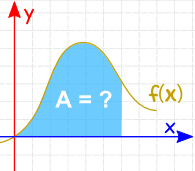我们可以把曲线下面的面积切成一片片，当这些片的宽度趋近零时，它们面积的和便是曲线下面的面积： 也有 积分法则 来帮我们求答案。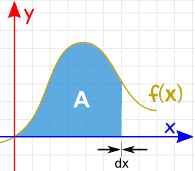## 记法

 "积分" 的符号像英语字母 "S" （源自英语 "Sum"（总和））：## 定积分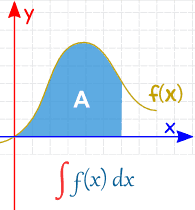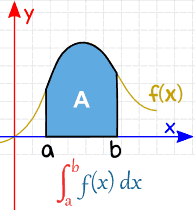不定积分 （没有指定值） 定积分 （从 a 到 b）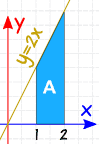### 例子：

2x dx 从 1 到 2 的定积分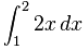• 在 x=1：2x dx = 12 + C
• 在 x=2：2x dx = 22 + C

(22 + C) − (12 + C)
22 + C − 12 − C
4 − 1 + C − C = 3

"C" 被消去…… 所以求定积分时我们可以不理 C。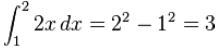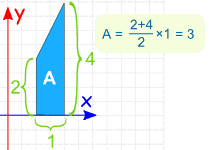（不赖！）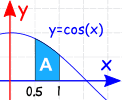### 例子：

cos(x) dx 从 0.5 到 1.0 的定积分：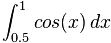（注意：x 的单位一定要是 弧度

C 可以不理（如上）：= sin(1) − sin(0.5) = 0.841…… − 0.479…… = 0.362……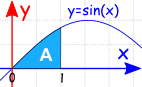### 例子：

sin(x) dx 从 0 到 1 的定积分：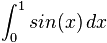−cos(1) = −0.540……= −cos(1) − (−cos(0)) = −0.540……− (−1) = 0.460……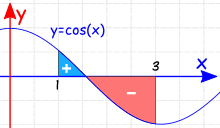### 例子：

cos(x) dx 从 1 到 3 的定积分：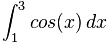= sin(3) − sin(1) = 0.141…… − 0.841…… = −0.700……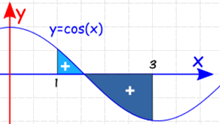### 例子：从 x = 1 到 x = 3，y = cos(x) 和 x轴 之间的面积是多少？

• 一部分是 x轴 上面的面积
• 一部分是 x轴 下面的面积

π/2
1
cos(x) dx = sin(π/2) − sin(1)
= 1 − 0.841……
= 0.159……
3
π/2
cos(x) dx = sin(3) − sin(π/2)
= 0.141... − 1
= −0.859……

## 连续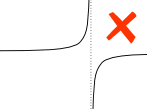### 例子：

ab 之间的垂直渐近线对定积分有影响。

## 属性

### 倒转区间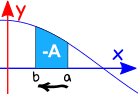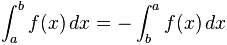### 零长度的区间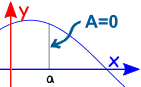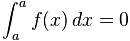### 区间相加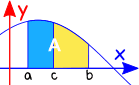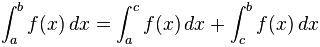## 总结

ab 的定积分是用在 b 的不定积分减去在 a 的不定积分。